# helper.c File Reference

General helper functions. More...

`#include <stdio.h>`
`#include <fcntl.h>`
`#include <unistd.h>`
`#include <ctype.h>`
`#include <dlfcn.h>`
`#include <netdb.h>`
`#include <time.h>`
`#include <sys/types.h>`
`#include <grp.h>`
`#include <poll.h>`
`#include <pwd.h>`
`#include <apr_file_io.h>`
`#include <apr_md5.h>`
`#include <subversion-1/svn_config.h>`
`#include "global.h"`
`#include "waa.h"`
`#include "est_ops.h"`
`#include "options.h"`
`#include "interface.h"`
`#include "checksum.h"`
`#include "helper.h"`
`#include "cache.h"`
`#include <iconv.h>`

Include dependency graph for helper.c: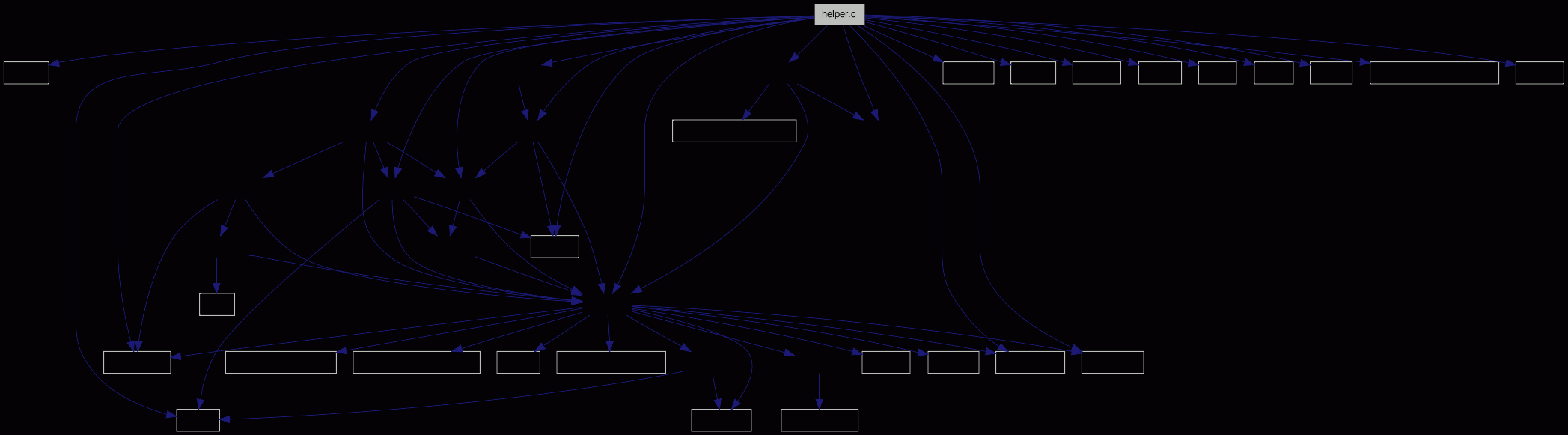Go to the source code of this file.

## Defines

#define STRING_LENGTH   (4096)

## Functions

int hlp___get_conv_handle (const char *from_charset, const char *to_charset, iconv_t *cd)
Initializer for i18n operations.
int hlp___do_convert (iconv_t cd, const char *from, char **to, int len)
Charset convert function.
int hlp___dummy_convert (const char *input, char **output, int len)
Dummy converter function.
int hlp__local2utf8 (const char *local_string, char **utf8_string, int len)
-.
int hlp__utf82local (const char *utf8_string, char **local_string, int len)
-.
void __attribute__ ((always_inline))
Small utility function to copy a system-defined struct stat into our own struct sstat_t - which is much smaller.
int hlp__lstat (const char *fn, struct sstat_t *st)
A wrapper for lstat().
int hlp__fstat (int fd, struct sstat_t *st)
A wrapper for fstat().
char * hlp__pathcopy (char *dst, int *len,...)
-.
int hlp__parse_rev (char *stg, char **eos, svn_revnum_t *rev)
-.
const char * hlp__get_grname (gid_t gid, char *not_found)
-.
const char * hlp__get_uname (uid_t uid, char *not_found)
-.
int hlp__get_uid (char *user, uid_t *uid, apr_pool_t *pool)
-.
int hlp__get_gid (char *group, gid_t *gid, apr_pool_t *pool)
-.
int hlp__string_from_filep (FILE *input, char **string, char **eos, int flags)
-.
int hlp__safe_print (FILE *output, char *string, int maxlen)
-.
int hlp___encoder_waiter (struct encoder_t *encoder)
select() loop for the encoder pipes.
svn_error_t * hlp___encode_write (void *baton, const char *data, apr_size_t *len)
Writer function for an encoder.
svn_error_t * hlp___encode_read (void *baton, char *data, apr_size_t *len)
svn_error_t * hlp___encode_close (void *baton)
void hlp___encode_filter_child (int pipe_in, int pipe_out, const char *path, const char *command)
Helper function.
int hlp__encode_filter (svn_stream_t *s_stream, const char *command, int is_writer, char *path, svn_stream_t **output, struct encoder_t **encoder_out, apr_pool_t *pool)
-.
int hlp__chrooter (void)
Checks for the needed environment variables, and does the chroot()ing if necessary.
int hlp___is_valid_env (char *env, char *path2cmp, int p2c_len, char **value, int *value_len)
Is the given environment valid for substitution? Returns a boolean - 0 means not valid, everything else ok.
int hlp__match_path_envs (struct estat *root)
Here we simply (try to) match the (few) environment variables against the entries, and set pointers for matching paths; so for displaying them it suffices to walk the tree up until an entry with `arg` set is found.
int hlp__format_path (struct estat *sts, char *wc_relative_path, char **output)
Can be in several formats; see Displaying paths.
char * hlp__rev_to_string (svn_revnum_t rev)
-.
int hlp__strncmp_uline_eq_dash (char *always_ul, char *other, int max)
-.
int hlp__is_special_property_name (const char *name)
-.
int hlp__stream_md5 (svn_stream_t *stream, unsigned char md5[APR_MD5_DIGESTSIZE])
-.
int hlp__delay (time_t start, enum opt__delay_e which)
Delays execution until the next second.
int hlp__rename_to_unique (char *fn, char *extension, const char **unique_name, apr_pool_t *pool)
-.
int hlp__get_svn_config (apr_hash_t **config)
-.
int hlp__strnalloc (int len, char **dest, const char const *source)
-.
int hlp__strmnalloc (int len, char **dest, const char const *source,...)
-.
int hlp__calloc (void *output, size_t nmemb, size_t count)
-.
int hlp__realloc (void *output, size_t size)
-.
char * hlp__get_word (char *input, char **word_start)
-.
int hlp__compare_string_pointers (const void *a, const void *b)

## Detailed Description

General helper functions.

Definition in file helper.c.

## Define Documentation

 #define STRING_LENGTH   (4096)

Definition at line 733 of file helper.c.

Referenced by hlp__string_from_filep().

## Function Documentation

 void __attribute__ ( (always_inline) ) ` [inline]`

Small utility function to copy a system-defined struct stat into our own struct sstat_t - which is much smaller.

Definition at line 297 of file helper.c.

 int hlp___do_convert ( iconv_t cd, const char * from, char ** to, int len ) ` [inline]`

Charset convert function.

Using a handle obtained with hlp___get_conv_handle() this function dynamically allocates some buffer space, and returns the converted data in it.

Parameters:
 cd A conversion handle from The source string to A pointer to the converted string. len The maximum number of input characters to translate.
If from is NULL, to is returned as NULL.

A few buffers are used round-robin, so that the caller need not free anything and the maximum memory usage is limited. Normally only 1 or 2 buffers are "active", eg. file name for a symlink and its destination, or source and destination for apply_textdelta.

The destination string is always terminated with a \0.

Note:
If there's an irreparable conversion error, we must not print (parts of) the strings. One or even both might not be suitable for printing on the current console - so we do not know what could happen.

Definition at line 87 of file helper.c.

Referenced by hlp__local2utf8(), and hlp__utf82local().

Here is the call graph for this function:Here is the caller graph for this function: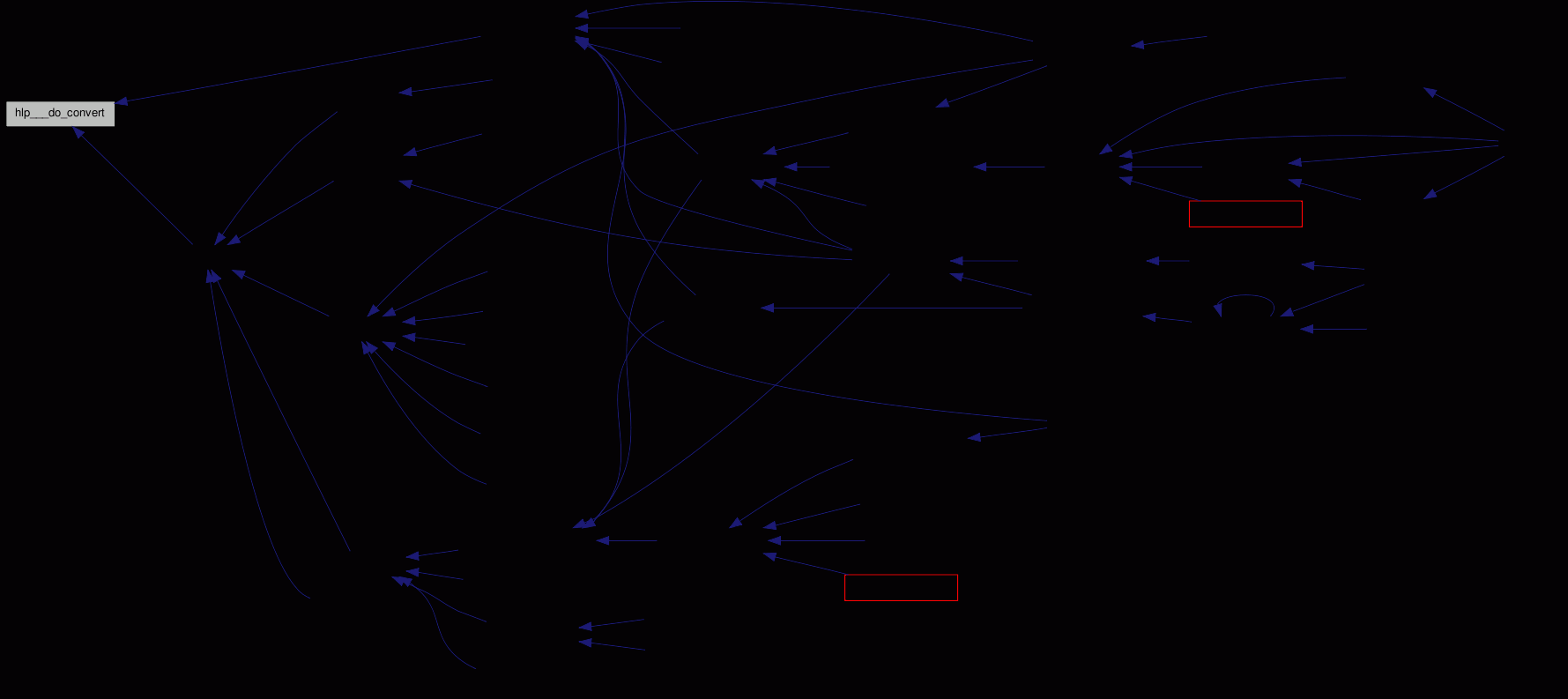int hlp___dummy_convert ( const char * input, char ** output, int len )

Dummy converter function.

We need to honor the length parameter; but this function XOR the hlp___do_convert() run, so only one cache is active.

If the length is given as `-1`, the original string is returned.

Definition at line 200 of file helper.c.

Referenced by hlp__local2utf8(), and hlp__utf82local().

Here is the call graph for this function:Here is the caller graph for this function: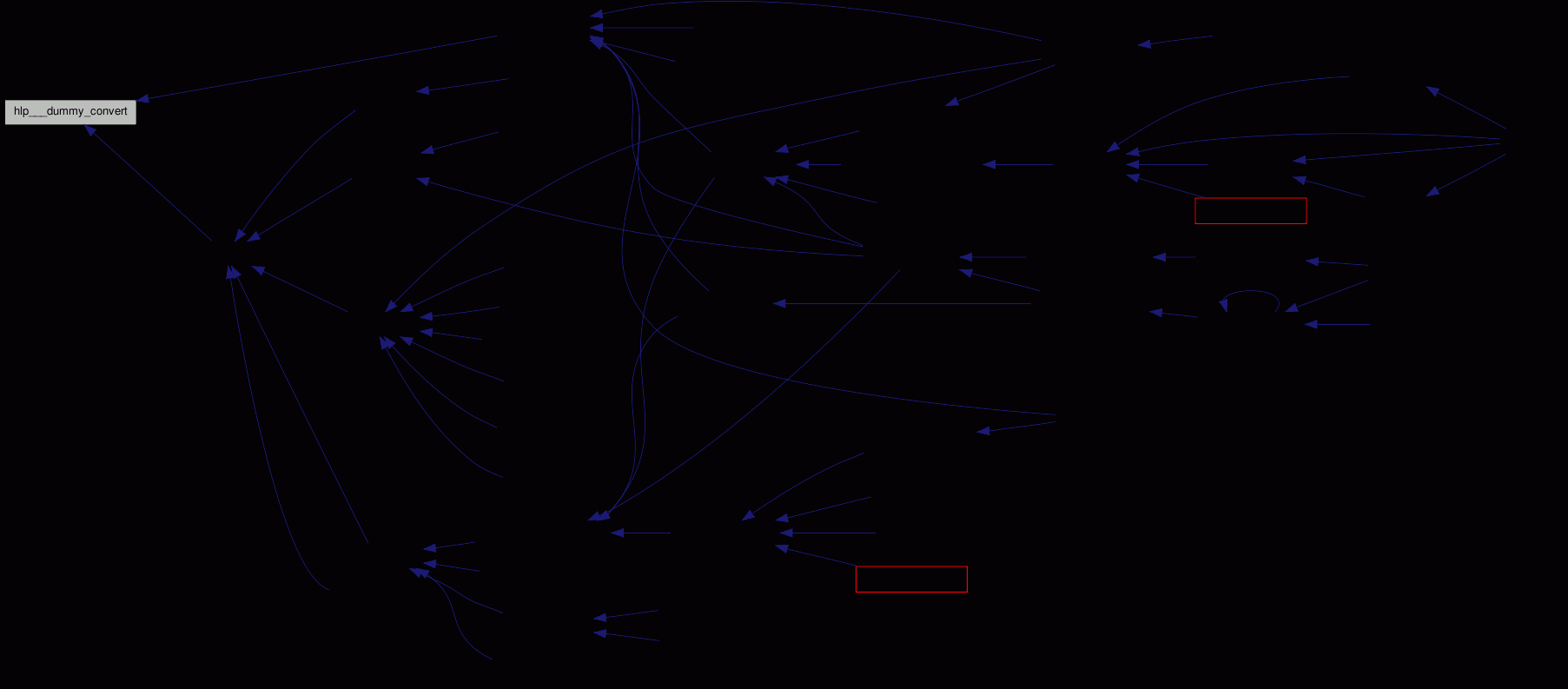svn_error_t* hlp___encode_close ( void * baton )

Definition at line 1154 of file helper.c.

Referenced by hlp__encode_filter().

Here is the call graph for this function:Here is the caller graph for this function: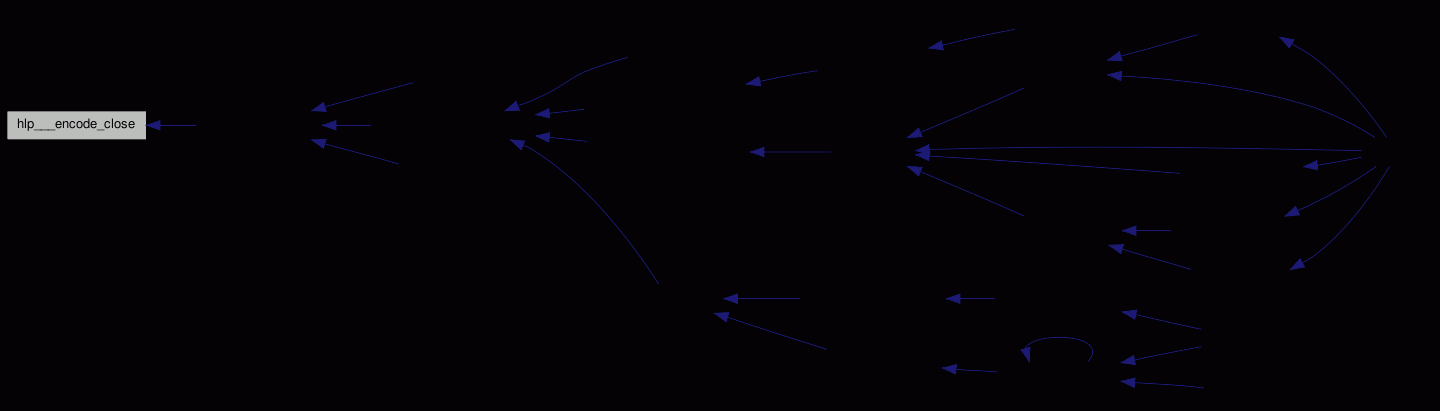void hlp___encode_filter_child ( int pipe_in, int pipe_out, const char * path, const char * command )

Helper function.

Could be marked `noreturn`.

Definition at line 1201 of file helper.c.

References FSVS_EXP_CURR_ENTRY, PATH_SEPARATOR, and STOPIF_CODE_ERR.

Referenced by hlp__encode_filter().

Here is the caller graph for this function: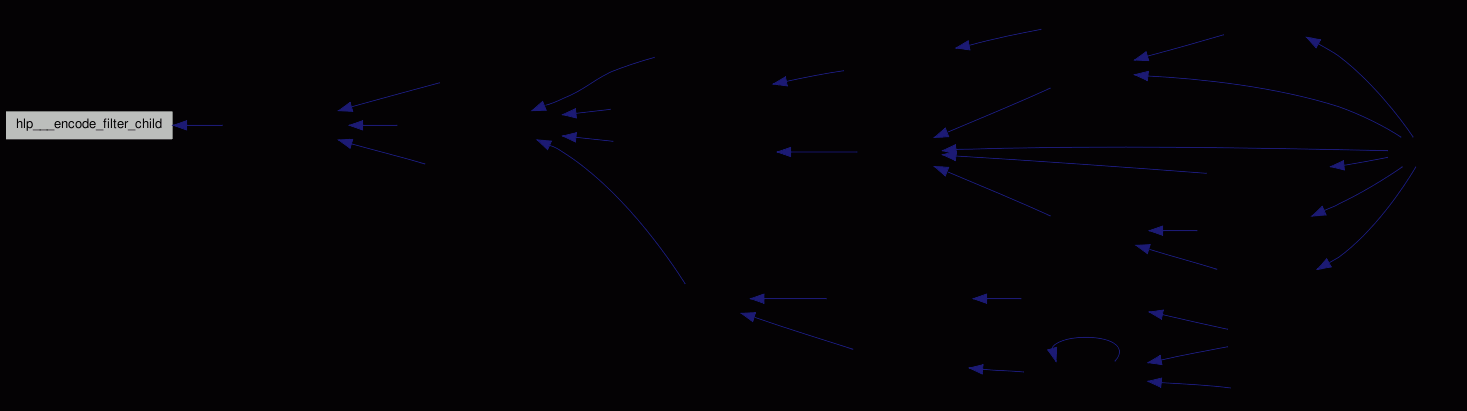svn_error_t* hlp___encode_read ( void * baton, char * data, apr_size_t * len )

A `svn_stream_t` reader gets as much data as it requested; a short read would be interpreted as EOF.

Definition at line 1030 of file helper.c.

Referenced by hlp__encode_filter().

Here is the call graph for this function: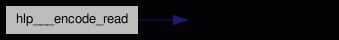Here is the caller graph for this function: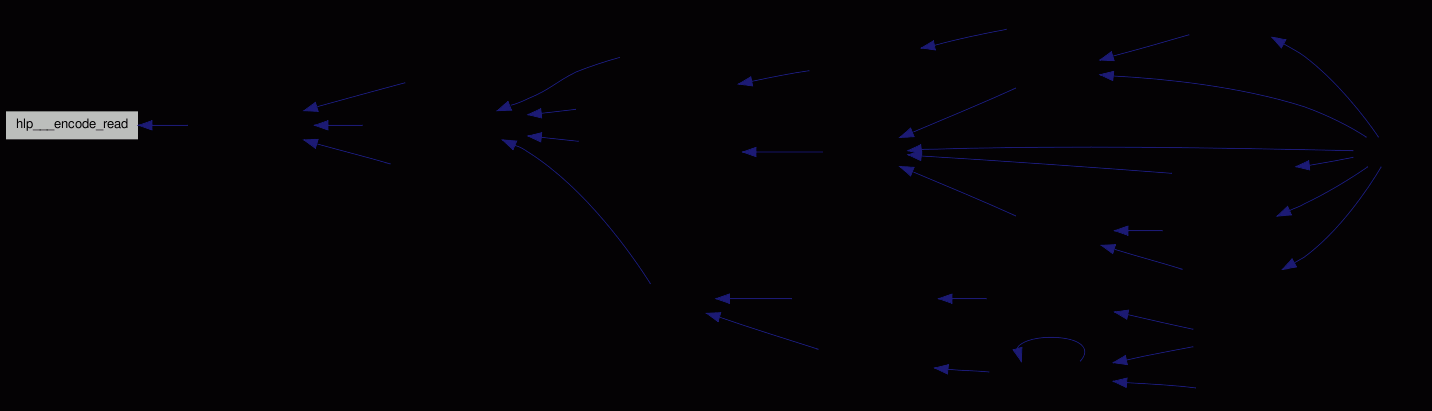svn_error_t* hlp___encode_write ( void * baton, const char * data, apr_size_t * len )

Writer function for an encoder.

We have to write the full buffer through the pipe before returning - possibly in some chunks.

Definition at line 919 of file helper.c.

Referenced by hlp___encode_close(), and hlp__encode_filter().

Here is the call graph for this function: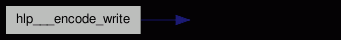Here is the caller graph for this function: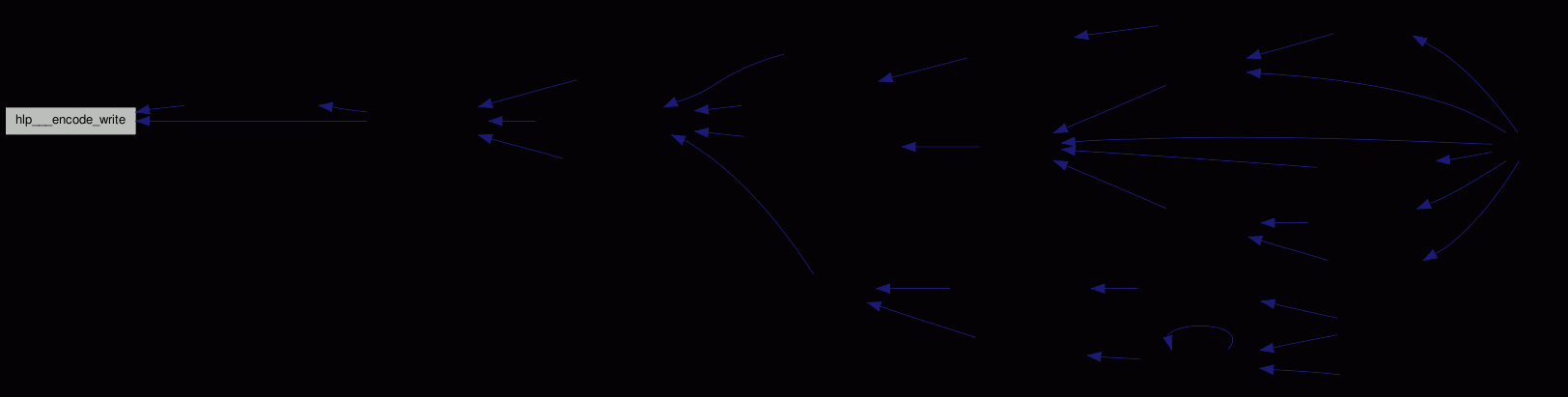int hlp___encoder_waiter ( struct encoder_t * encoder )

select() loop for the encoder pipes.

As we can always get/put data from/to the associated stream, the only rate limiter is the child process - how fast it can take/give data.

So we have to poll.

Definition at line 886 of file helper.c.

References encoder_t::is_writer, encoder_t::pipe_in, encoder_t::pipe_out, and STOPIF_CODE_ERR.

Here is the caller graph for this function: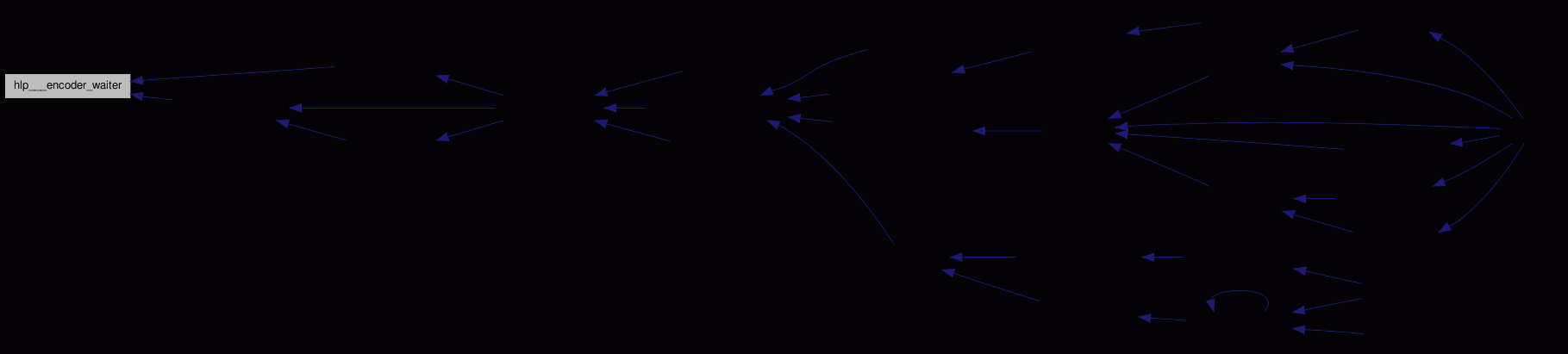int hlp___get_conv_handle ( const char * from_charset, const char * to_charset, iconv_t * cd )

Initializer for i18n operations.

Parameters:
 from_charset Source charset to_charset Destination charset. cd iconv-handle

Definition at line 48 of file helper.c.

References STOPIF_CODE_ERR.

Referenced by hlp__local2utf8(), and hlp__utf82local().

Here is the caller graph for this function: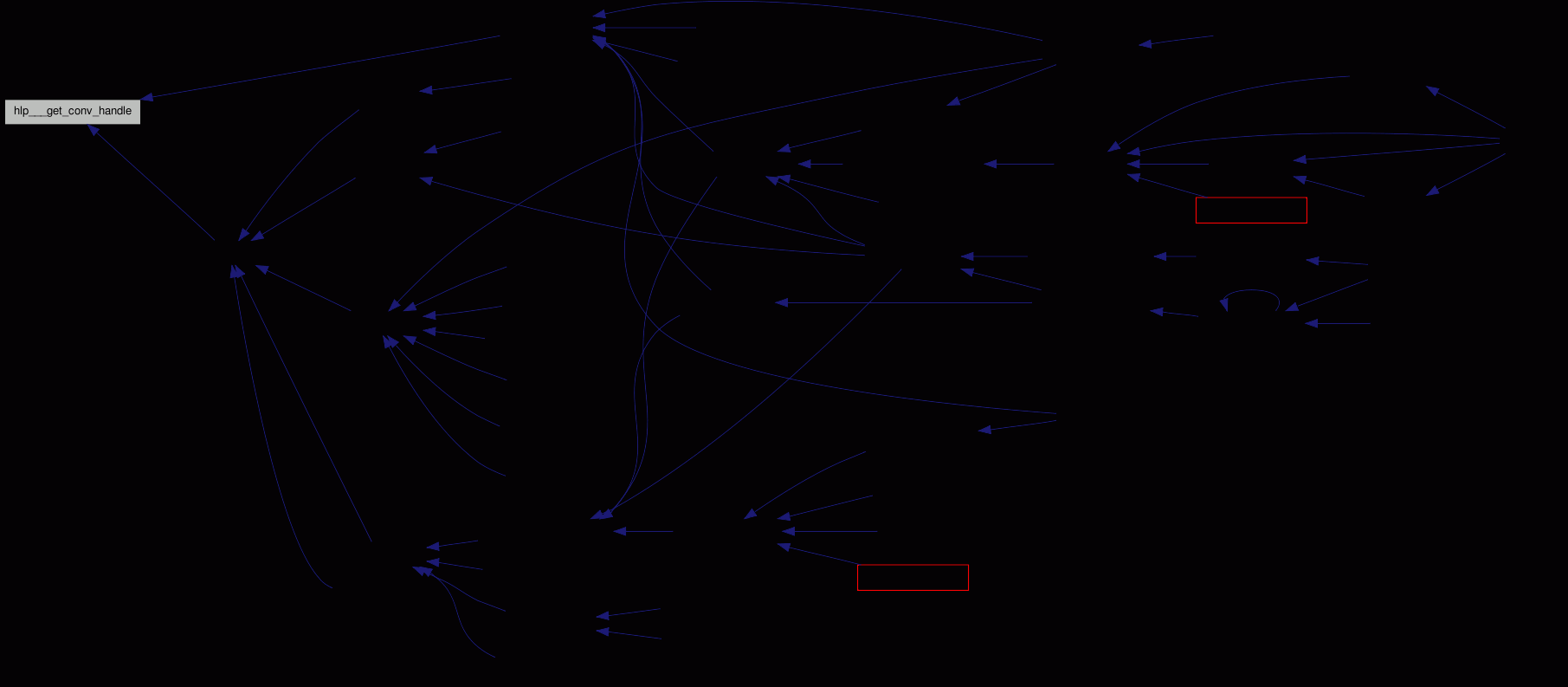int hlp___is_valid_env ( char * env, char * path2cmp, int p2c_len, char ** value, int * value_len ) ` [inline]`

Is the given environment valid for substitution? Returns a boolean - 0 means not valid, everything else ok.

It is valid, if

• The name starts with `WC`
• The first p2c_len characters of path2cmp match with the value. If `p2c_len` equals `-1` the length of the path in the environment variable is taken.

If value_len is not `NULL`, it get set to the length of the path in the environment variable.

Definition at line 1474 of file helper.c.

References PATH_SEPARATOR.

Referenced by hlp__format_path(), and hlp__match_path_envs().

Here is the caller graph for this function: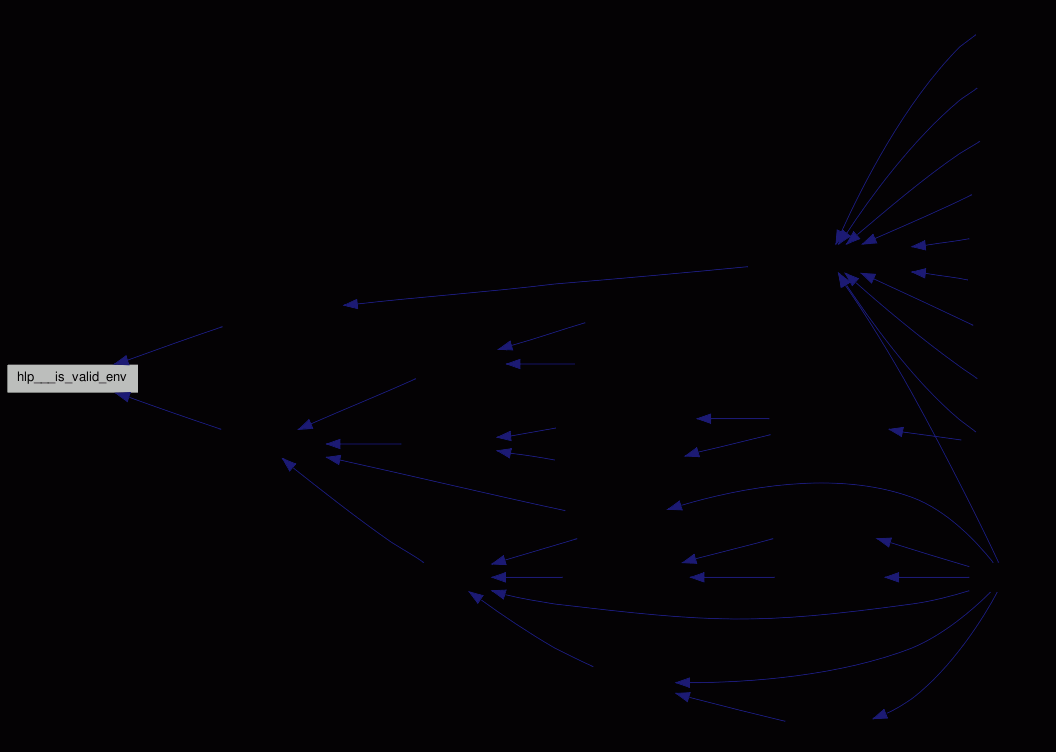int hlp__calloc ( void * output, size_t nmemb, size_t count )

-.

Error returning `calloc()`; uses (void**) output.

That is not defined in terms of `hlp__alloc()`, because glibc might do some magic to get automagically 0-initialized memory (like mapping `/dev/zero`).

Definition at line 1981 of file helper.c.

References STOPIF_CODE_ERR.

Here is the caller graph for this function: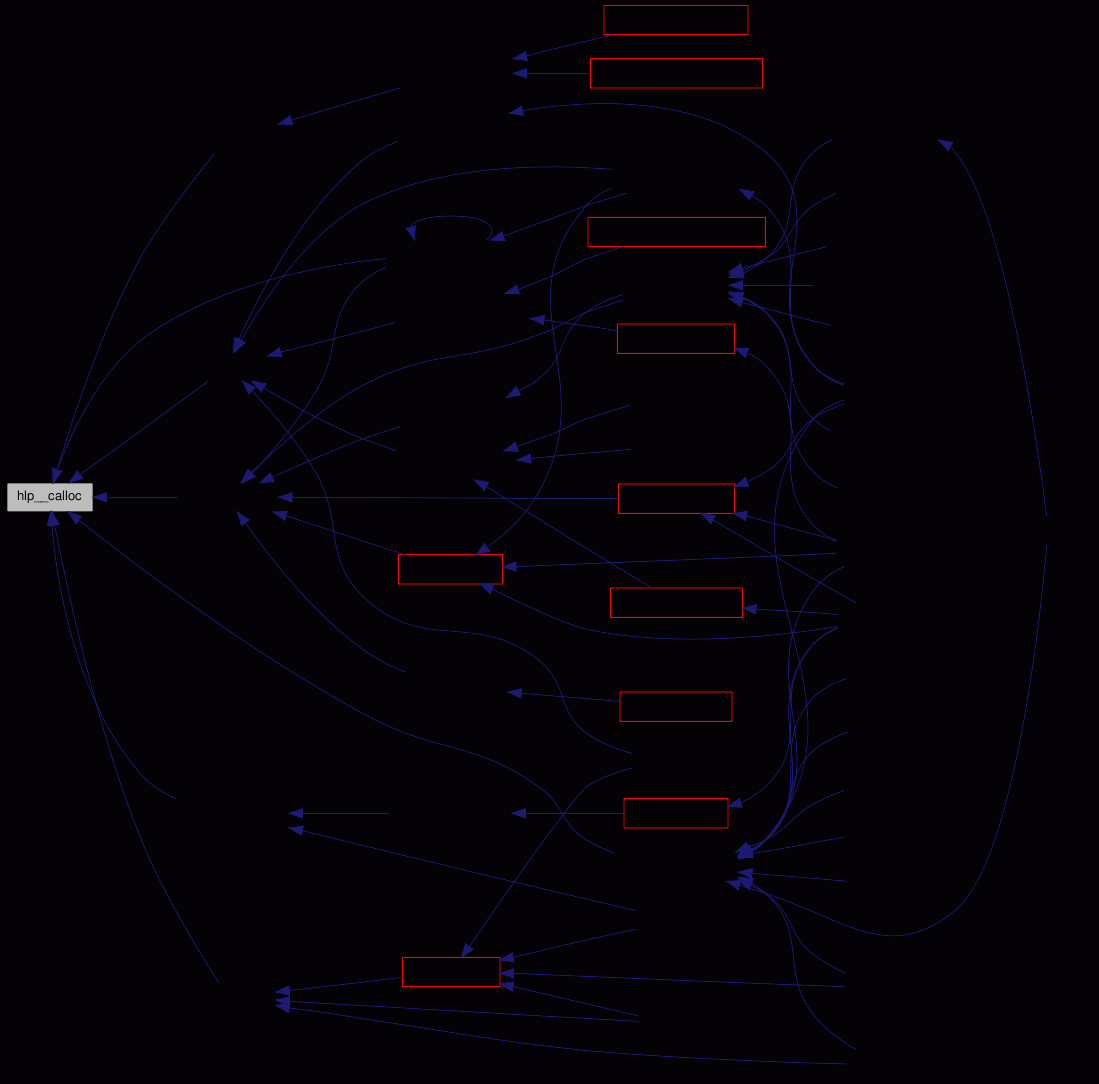int hlp__chrooter ( void )

Checks for the needed environment variables, and does the chroot()ing if necessary.

Chroot helper function.

Definition at line 1363 of file helper.c.

References CHROOTER_CWD_ENV, CHROOTER_LIBS_ENV, CHROOTER_ROOT_ENV, DEBUGP, filename, and STOPIF_CODE_ERR.

Referenced by main().

Here is the caller graph for this function: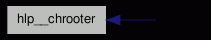int hlp__compare_string_pointers ( const void * a, const void * b )

Definition at line 2068 of file helper.c.

Here is the caller graph for this function: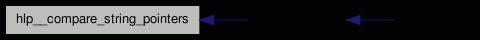int hlp__delay ( time_t start, enum opt__delay_e which )

Delays execution until the next second.

Delay until time wraps.

Needed because of filesystem granularities; FSVS only stores seconds, not more.

Definition at line 1841 of file helper.c.

References DEBUGP, and OPT__DELAY.

Referenced by ci__work(), co__work(), delay__work(), rev__work(), and up__work().

Here is the caller graph for this function: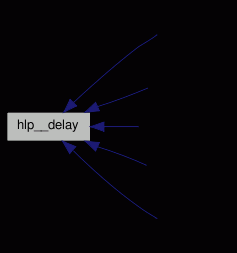int hlp__encode_filter ( svn_stream_t * s_stream, const char * command, int is_writer, char * path, svn_stream_t ** output, struct encoder_t ** encoder_out, apr_pool_t * pool )

-.

Encode `svn_stream_t` filter.

For is_writer data gets written into s_stream, gets piped as `STDIN` into command, and the resulting `STDOUT` can be read back with output; if is_writer is zero, data must be read from output to get processed.

After finishing the returned pointer *encoder_out can be used to retrieve the MD5.

*encoder_out must not be `free()d`; delta editors that store data locally get driven by the subversion libraries, and the only place where we can be sure that it's no longer in use is when the streams get closed. Therefore the `free()ing` has to be done in hlp___encode_close(); callers that want to get the final MD5 can set the `encoder->output_md5` pointer to the destination address.

Definition at line 1293 of file helper.c.

Referenced by ci__nondir(), rev__get_text_to_stream(), and up__apply_textdelta().

Here is the call graph for this function: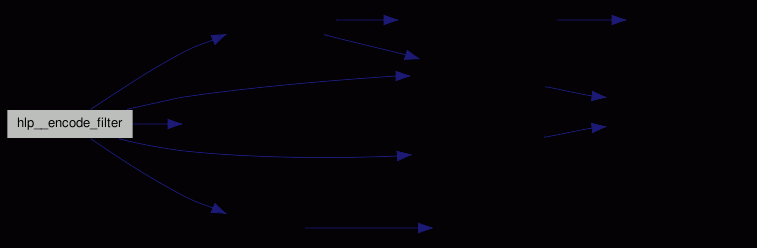Here is the caller graph for this function: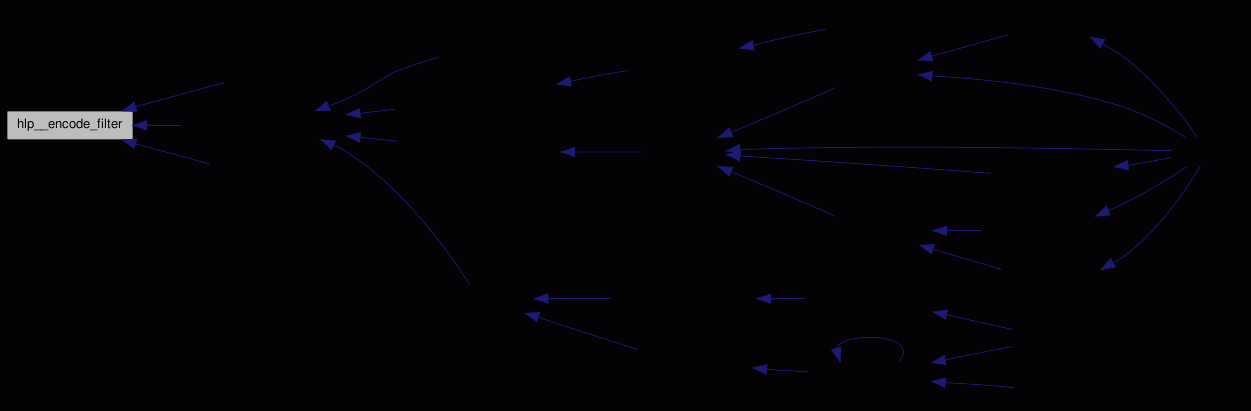int hlp__format_path ( struct estat * sts, char * wc_relative_path, char ** output )

Can be in several formats; see Displaying paths.

Return a path that gets displayed for the user.

Todo:
Build the `wc_relative_path` only if necessary - remove the parameter from the caller chains.

Todo:
We should set it beginning from a command line parameter, if we have one. Preferably the nearest one ...

Definition at line 1569 of file helper.c.

Referenced by cm___match(), df___repos_repos(), df__do_diff(), and st__print_status().

Here is the call graph for this function: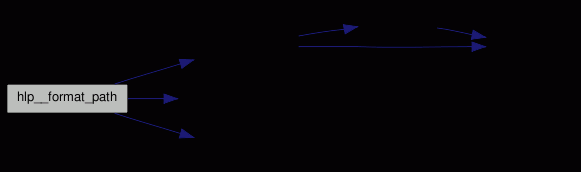Here is the caller graph for this function: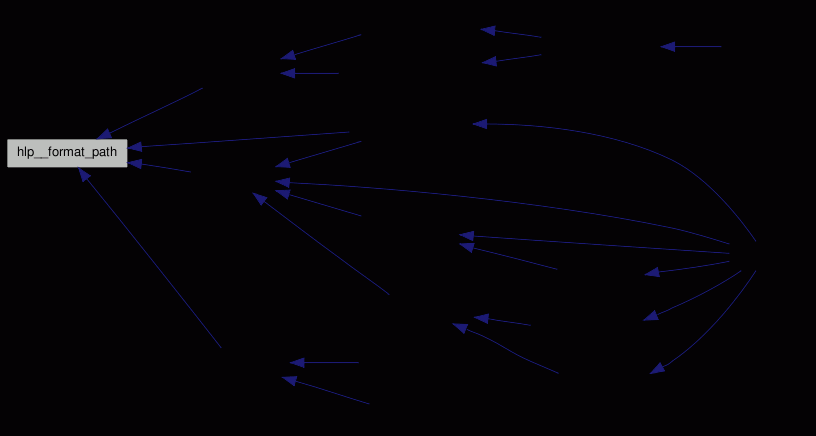int hlp__get_gid ( char * group, gid_t * gid, apr_pool_t * pool )

-.

Find a `GID` by group name, cached.

Uses a simple hash function.

Definition at line 705 of file helper.c.

Referenced by up__parse_prop().

Here is the call graph for this function: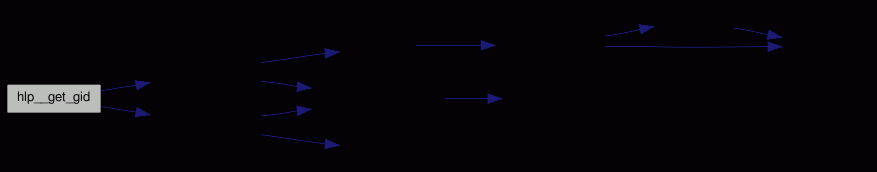Here is the caller graph for this function: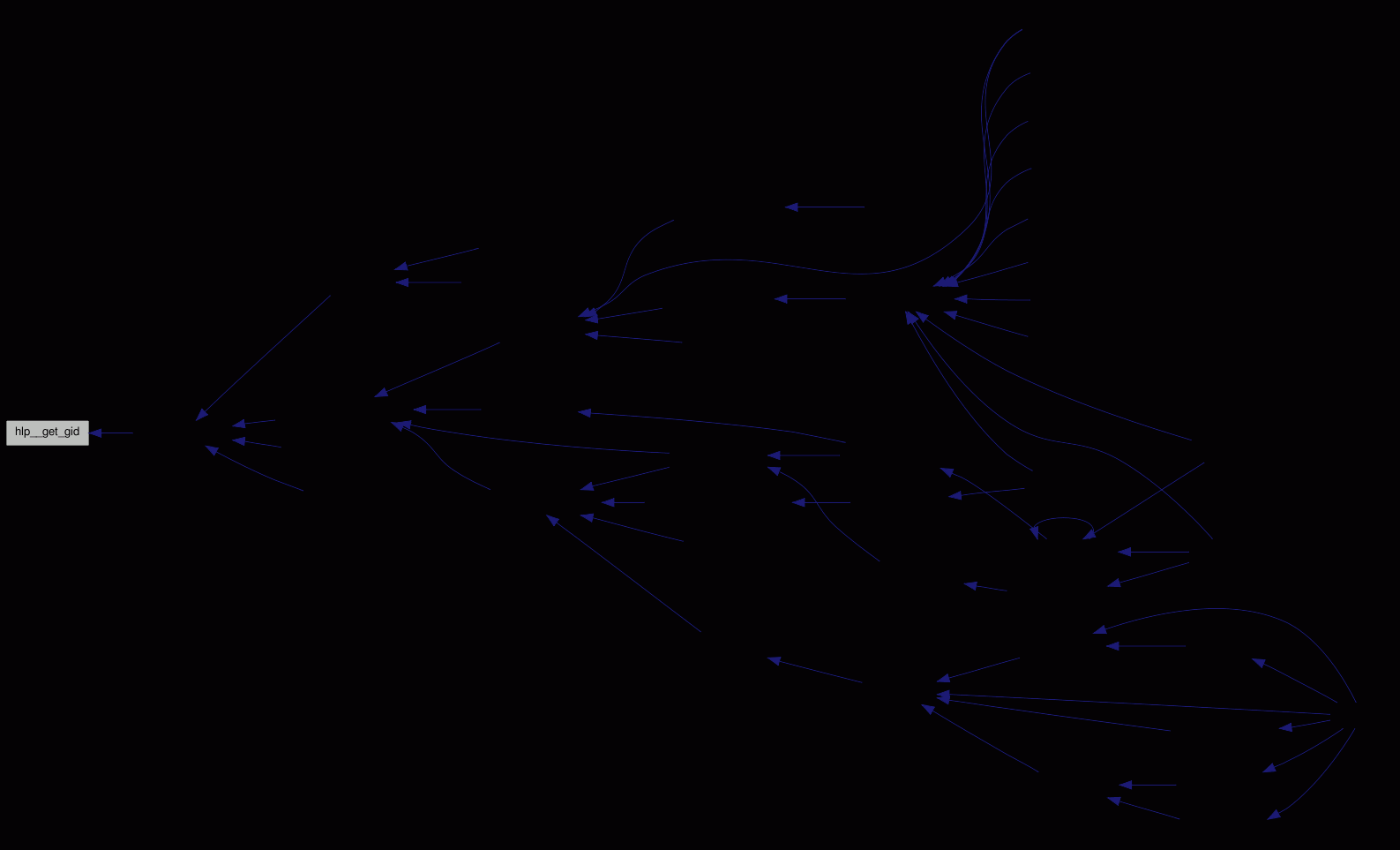const char* hlp__get_grname ( gid_t gid, char * not_found )

-.

Returns the name of the given group.

Has a few buffers for these operations.

The cache is statically allocated, as we cannot return `ENOMEM`.

If we cannot store a cache during querying, we'll return the value, but forget that we already know it.

Todo:
Keep most-used?

Definition at line 631 of file helper.c.

References CACHE_DEFAULT, cch__add(), cch__find(), and cache_t::max.

Referenced by ci___set_props(), df__do_diff(), and st__print_entry_info().

Here is the call graph for this function:Here is the caller graph for this function: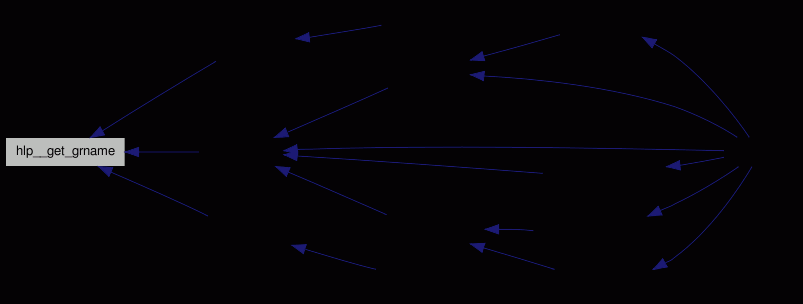int hlp__get_svn_config ( apr_hash_t ** config )

-.

Reads the subversion config file(s), found by Configuration directory for the subversion libraries.

Caches the result, so that the configuration is only fetched a single time.

Definition at line 1907 of file helper.c.

References global_pool, OPT__CONFIG_DIR, and STOPIF_SVNERR.

Referenced by cb__init(), and url__open_session().

Here is the caller graph for this function: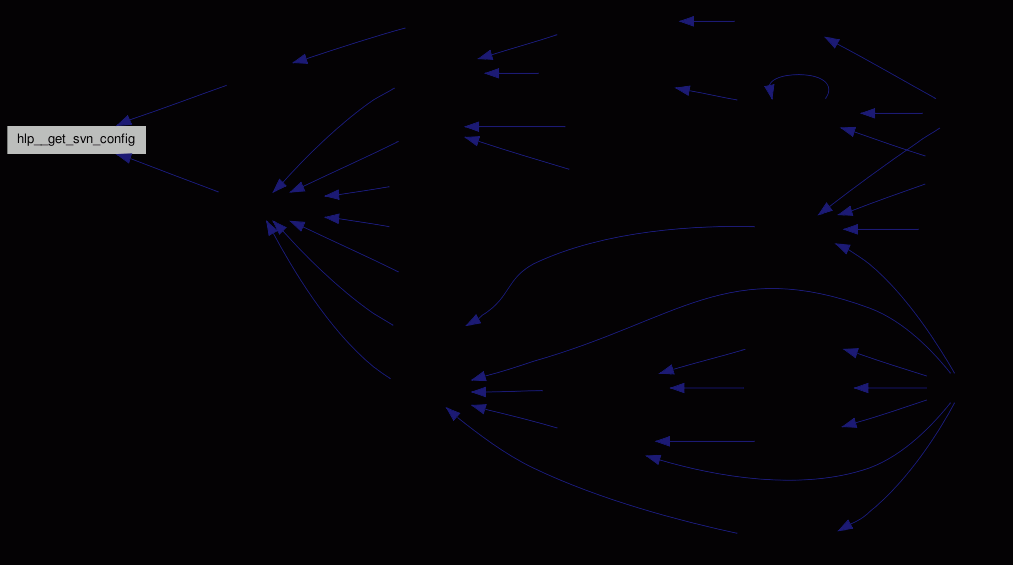int hlp__get_uid ( char * user, uid_t * uid, apr_pool_t * pool )

-.

Find a `UID` by user name, cached.

Uses a simple hash function.

Definition at line 673 of file helper.c.

Referenced by up__parse_prop().

Here is the call graph for this function: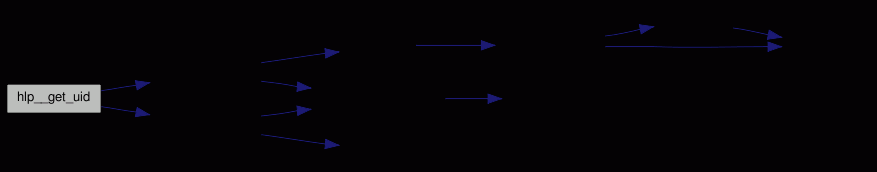Here is the caller graph for this function: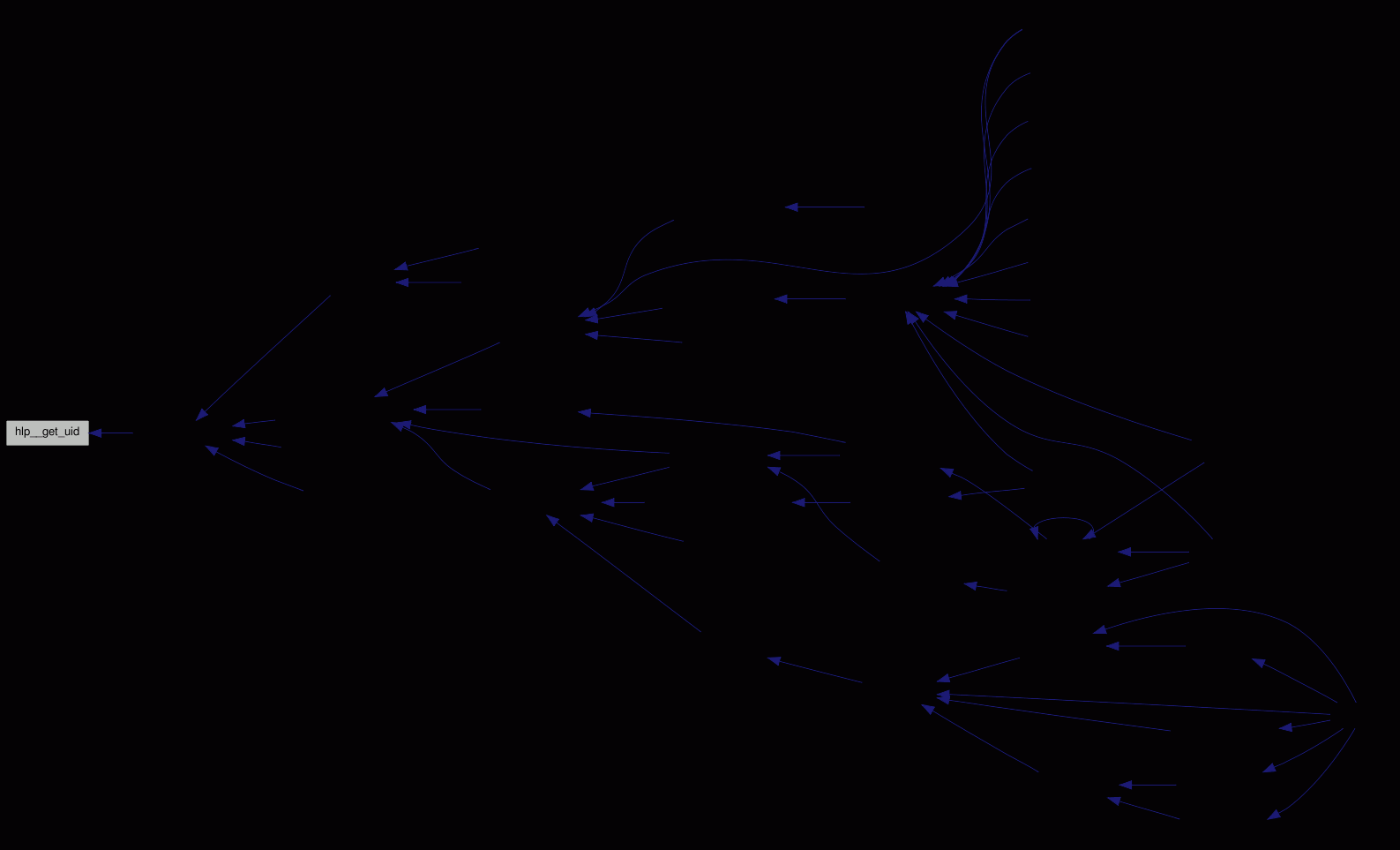const char* hlp__get_uname ( uid_t uid, char * not_found )

-.

Returns the name of the given user.

Has a few buffers for these operations; the cache is statically allocated, as we cannot return `ENOMEM`.

If we cannot store a cache during querying, we'll return the value, but forget that we already know it.

Definition at line 655 of file helper.c.

References CACHE_DEFAULT, cch__add(), cch__find(), and cache_t::max.

Referenced by ci___set_props(), df__do_diff(), and st__print_entry_info().

Here is the call graph for this function:Here is the caller graph for this function: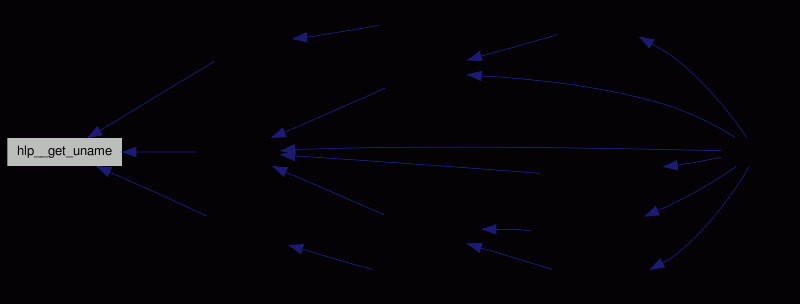char* hlp__get_word ( char * input, char ** word_start )

-.

Stores the first non-whitespace character position from input in word_start, and returns the next whitespace position in word_end.

Definition at line 2015 of file helper.c.

References hlp__skip_ws().

Here is the call graph for this function: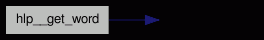Here is the caller graph for this function:int hlp__is_special_property_name ( const char * name )

-.

name is a subversion internal property.

Definition at line 1790 of file helper.c.

Referenced by ci___send_user_props(), and up__parse_prop().

Here is the caller graph for this function: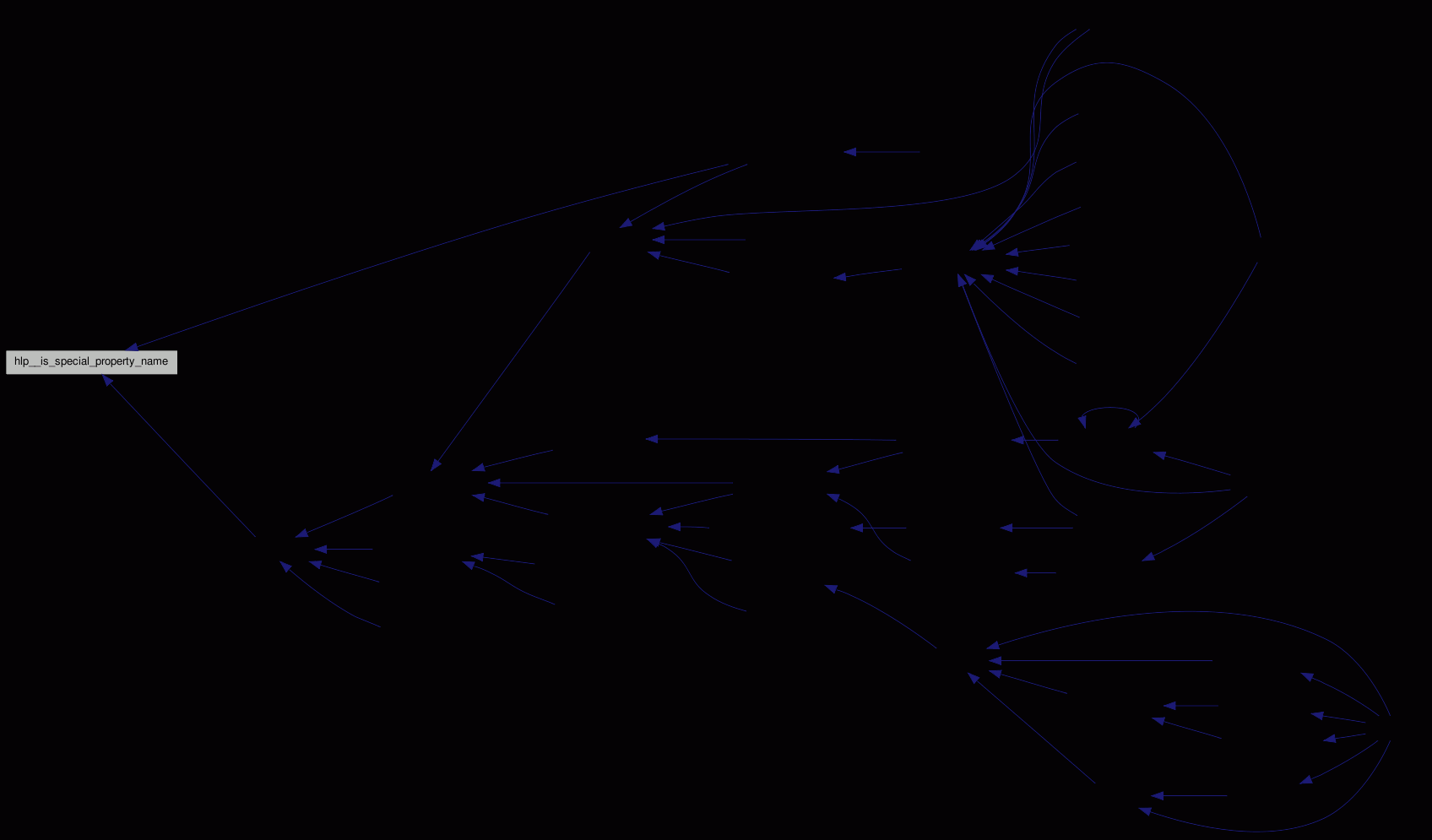int hlp__local2utf8 ( const char * local_string, char ** utf8_string, int len )

-.

Converts a string with local encoding to UTF8, suitable for storage in the repository.

If len `==-1`, a `strlen()` is done.

Definition at line 227 of file helper.c.

Here is the call graph for this function: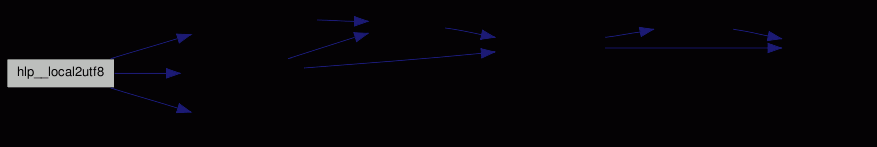Here is the caller graph for this function: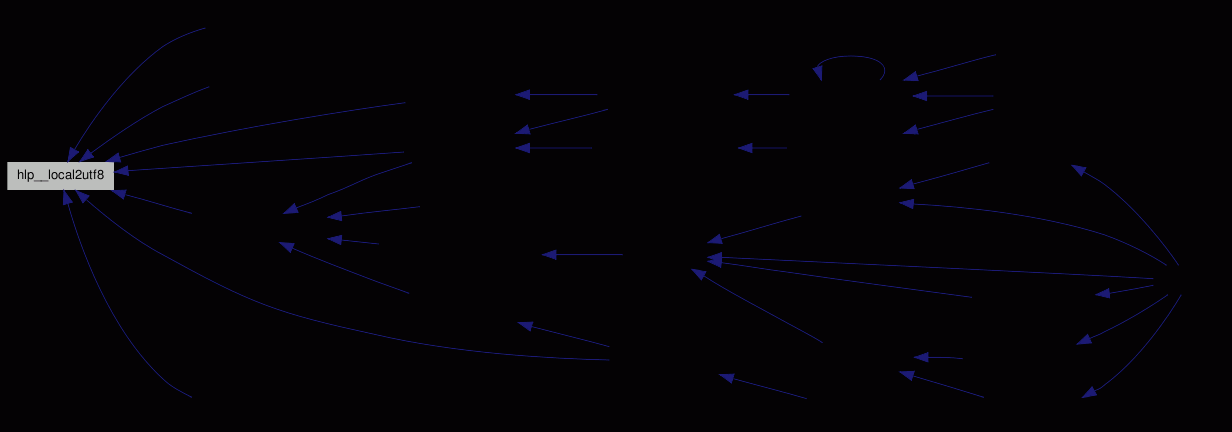int hlp__match_path_envs ( struct estat * root )

Here we simply (try to) match the (few) environment variables against the entries, and set pointers for matching paths; so for displaying them it suffices to walk the tree up until an entry with `arg` set is found.

Distribute the environment variables on the loaded entries.

Definition at line 1508 of file helper.c.

Here is the call graph for this function: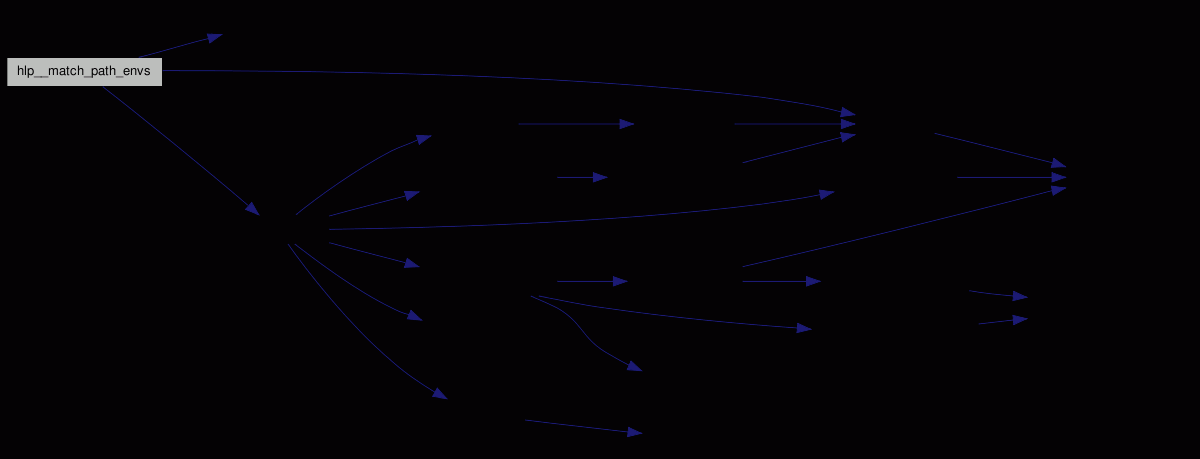Here is the caller graph for this function:int hlp__parse_rev ( char * stg, char ** eos, svn_revnum_t * rev )

-.

Parses a string to a revision number.

Normally a number; currently a special case is recogniced, namely `HEAD` .

If the parameter `eos` is not `NULL`, it gets set to the character behind the parsed part, which is ignored. If it is `NULL`, the string must end here.

Definition at line 591 of file helper.c.

References opt_target_revision, and STOPIF_CODE_ERR.

Referenced by main(), url__mark_todo(), and url__parse().

Here is the caller graph for this function: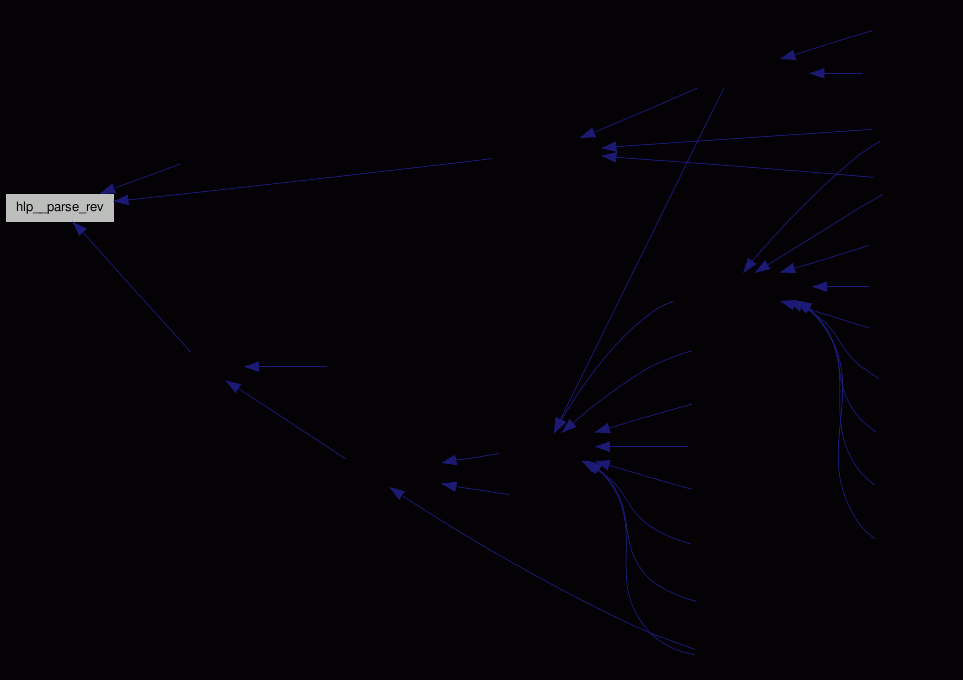char* hlp__pathcopy ( char * dst, int * len, ... )

-.

A function like strcpy, but cleaning up paths.

Parameters:
 dst A target buffer len An optional length-saving field ... A `NULL` -terminated list of pointers, which will be concatenated.
Returns:
dst as target buffer.
This works like `strcpy`, but removes sequences like `/./` and `//`.

Warning:
The buffer dst will be overwritten and has to have enough space!
If the first path has no PATH_SEPARATOR as first character, the start_path is used as beginning - so that always full paths are returned.

Used for cases where we cannot run realpath() (as this follows symlinks, something we don't want), but need to take care of some strange pathnames.

Note:
Not as easy as it sounds - try to do cases like `a//..//.///b/c//`.
For people reading JoelOnSoftware and related pages - yes, this is cheating, but it works and is fast :-).

Definition at line 438 of file helper.c.

References DEBUGP, PATH_SEPARATOR, start_path, and start_path_len.

Here is the caller graph for this function: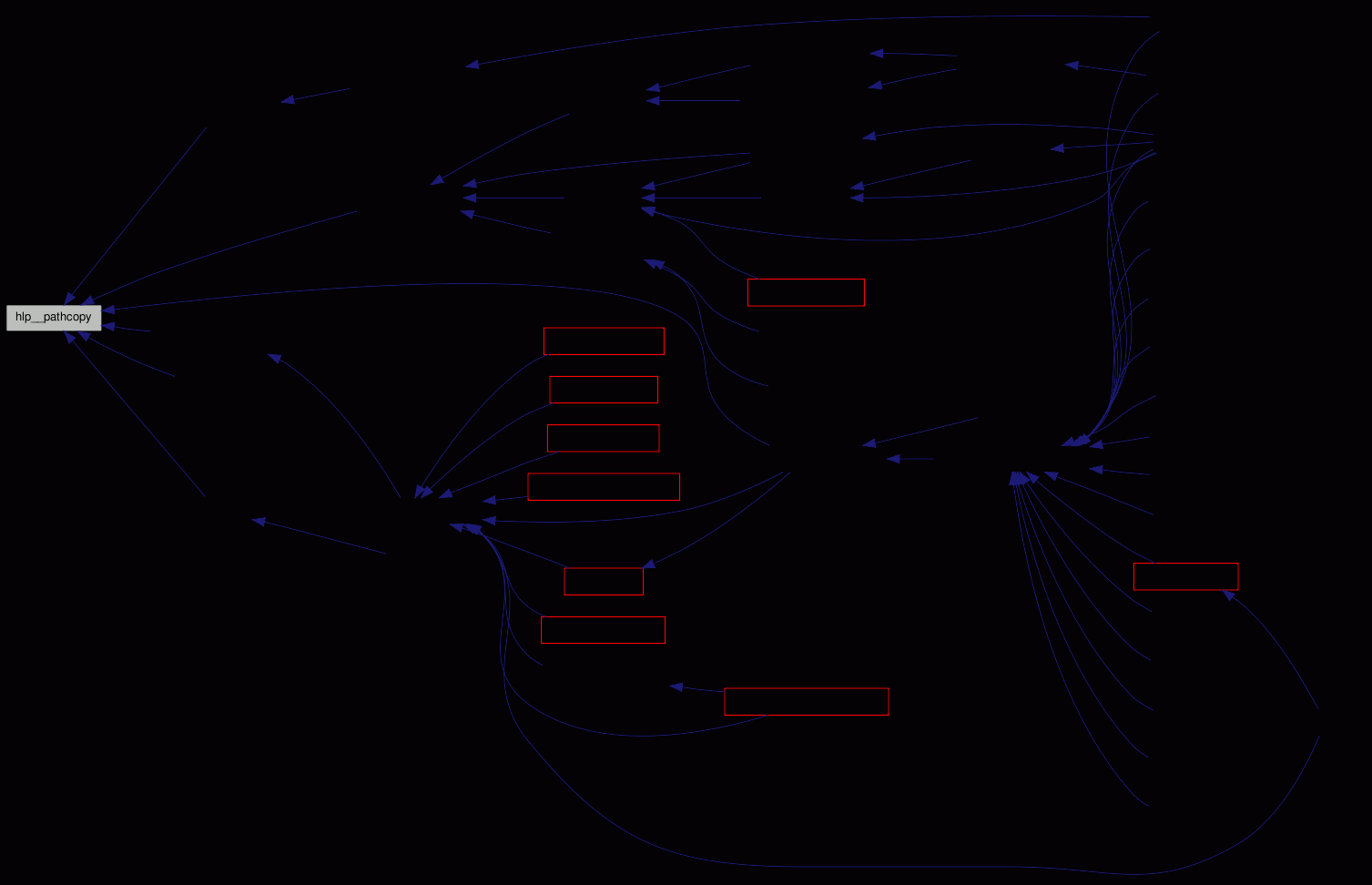int hlp__realloc ( void * output, size_t size )

-.

Reallocate the (void**) output.

Definition at line 1997 of file helper.c.

References STOPIF_CODE_ERR.

Here is the caller graph for this function: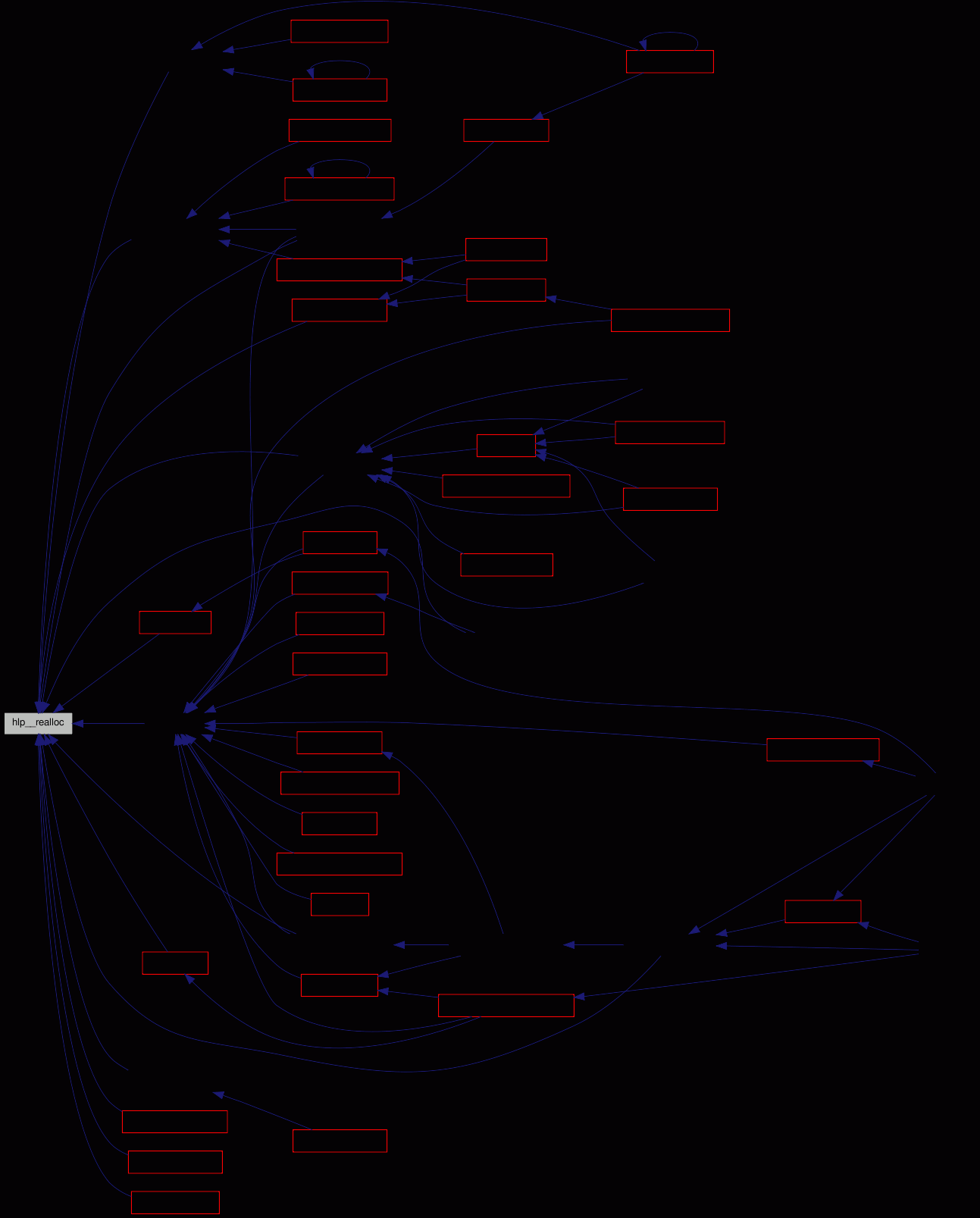int hlp__rename_to_unique ( char * fn, char * extension, const char ** unique_name, apr_pool_t * pool )

-.

Renames a local file to something like .mine.

We could either generate a name ourself, or just use this function - and have in mind that we open and close a file, just to overwrite it immediately.

But by using that function we get the behaviour that subversion users already know.

Definition at line 1864 of file helper.c.

References DEBUGP, STOPIF, and STOPIF_SVNERR.

Referenced by rev___undo_change().

Here is the caller graph for this function:char* hlp__rev_to_string ( svn_revnum_t rev )

-.

Returns a string describing the revision number.

Can be non-numeric, like `HEAD`.

Definition at line 1735 of file helper.c.

References BUG_ON.

Here is the caller graph for this function: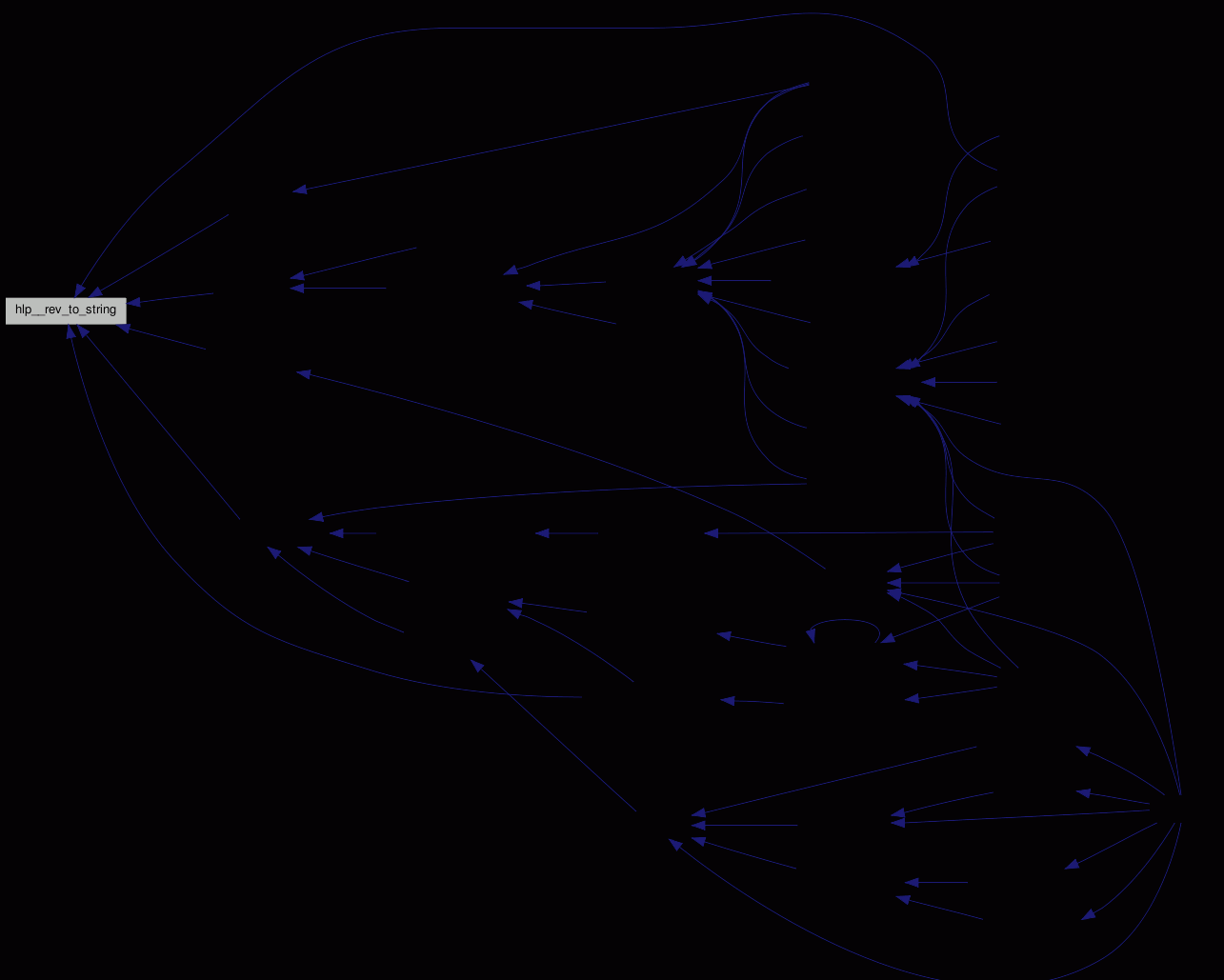int hlp__safe_print ( FILE * output, char * string, int maxlen )

-.

Print the given data to output, safely converting special characters to codes like `\x1e`.

Some special values are recogniced - eg. `\r`, `\n`.

Definition at line 829 of file helper.c.

References STOPIF_CODE_EPIPE.

Referenced by prp__l_work().

Here is the caller graph for this function: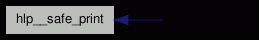int hlp__stream_md5 ( svn_stream_t * stream, unsigned char md5[APR_MD5_DIGESTSIZE] )

-.

Reads all data from stream and drops it.

md5, if not `NULL`, must point to at least MD5_DIGEST_LENGTH bytes.

Definition at line 1805 of file helper.c.

References DEBUGP, hlp__alloc(), estat::md5, STOPIF, and STOPIF_SVNERR.

Referenced by ci__nondir().

Here is the call graph for this function: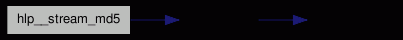Here is the caller graph for this function: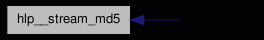int hlp__string_from_filep ( FILE * input, char ** string, char ** eos, int flags )

-.

Reads a string from the given `input` into the (self-managed) buffer string, removes \r and/or \n at the end, and depending on flags strips whitespace or comments.

Returns 0 for success, `EOF` for no more data.

Empty lines (only whitespace) are ignored (but counted).

If no_ws is set, the returned pointer has whitespace at beginning and end removed; `\r` and `\n` at the end are always removed.

Only a single statically allocated buffer is used, so the line has to be copied if needed over several invocations.

eos is set to the last non-whitespace character in the line.

Definition at line 746 of file helper.c.

Here is the call graph for this function:Here is the caller graph for this function:int hlp__strmnalloc ( int len, char ** dest, const char const * source, ... )

-.

Like `hlp__strnalloc`, but concatenates strings until a `NULL` is found.

Definition at line 1945 of file helper.c.

References BUG_ON, hlp__alloc(), and STOPIF.

Referenced by ci__getmsg(), ign___init_pattern_into(), and ign___load_group().

Here is the call graph for this function: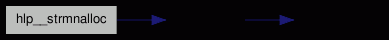Here is the caller graph for this function: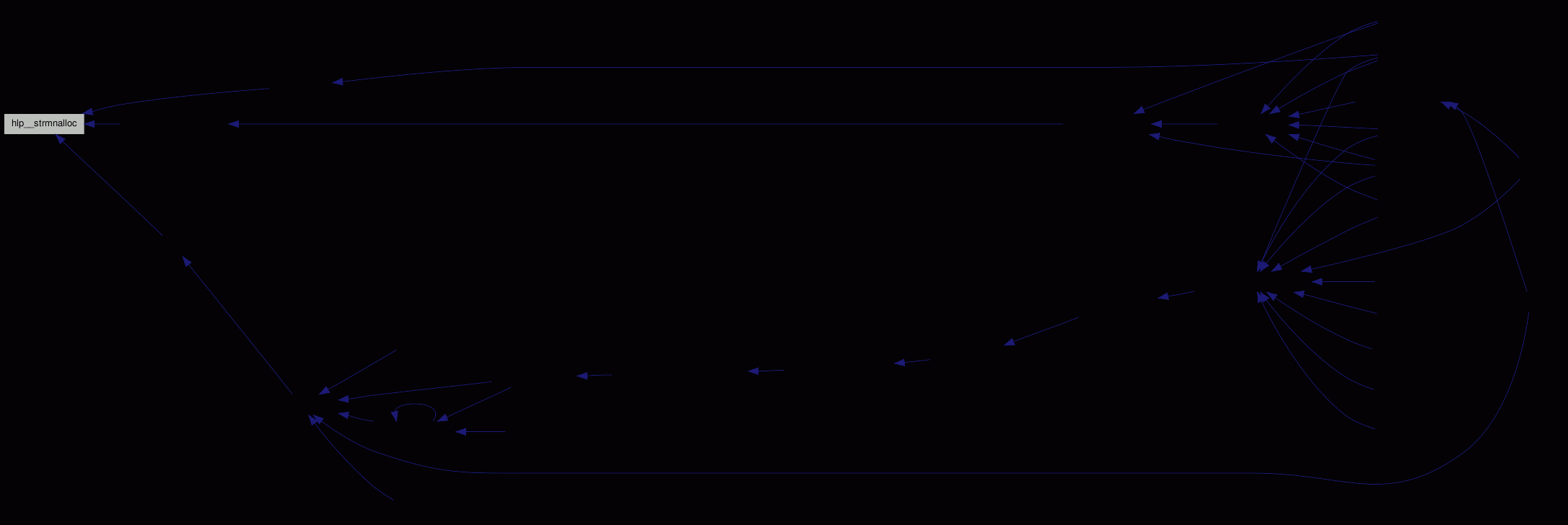int hlp__strnalloc ( int len, char ** dest, const char const * source )

-.

Allocates a buffer in *dest, and copies source.

If source is not `NULL` len bytes are copied. The buffer is always `\0` terminated.

Definition at line 1930 of file helper.c.

References hlp__alloc(), and STOPIF.

Here is the call graph for this function: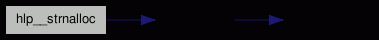Here is the caller graph for this function: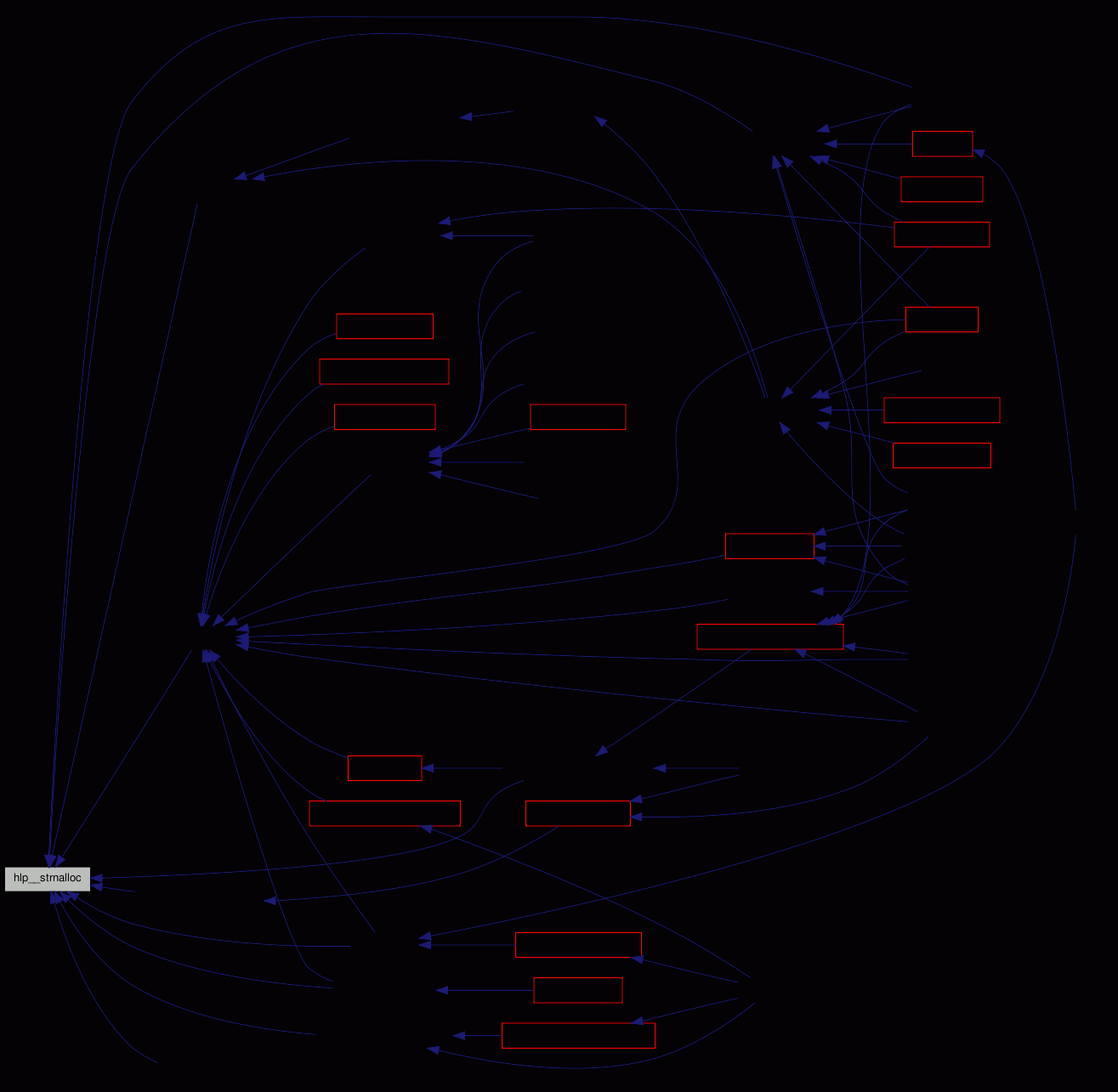int hlp__strncmp_uline_eq_dash ( char * always_ul, char * other, int max )

-.

Function to compare two strings for max bytes, but treating `'-'` and `'_'` as equal.

If max<0, the comparision is done until the `\0`. max is the maximum number of characters to compare; the result is always `equal` (`==0`), if `max==0`.

Not useable for lesser/greater compares.

Definition at line 1762 of file helper.c.

Referenced by opt__parse().

Here is the caller graph for this function: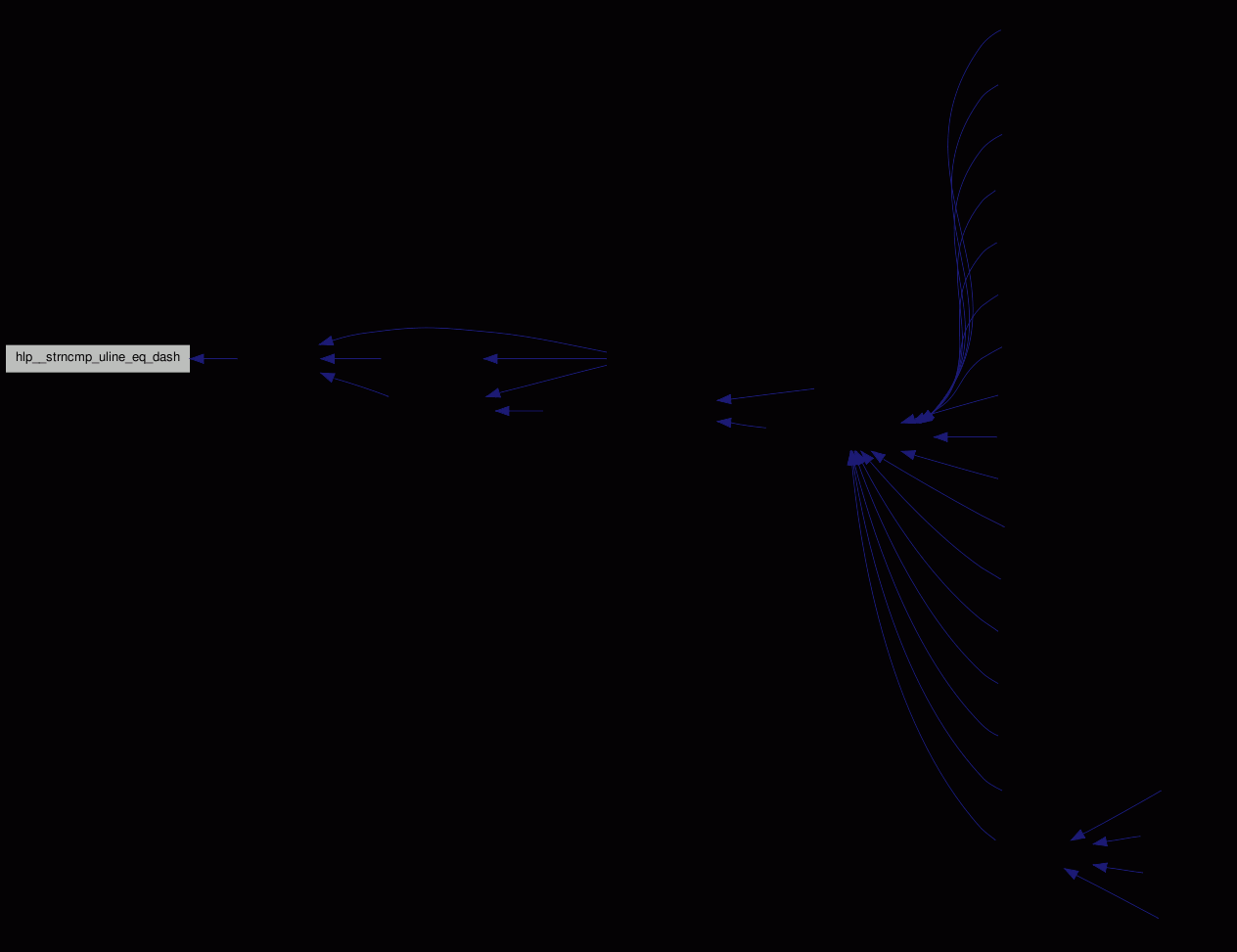int hlp__utf82local ( const char * utf8_string, char ** local_string, int len )

-.

Converts an UTF8 string to local encoding, to make it printable on the current console.

If len `==-1`, a `strlen()` is done.

Definition at line 255 of file helper.c.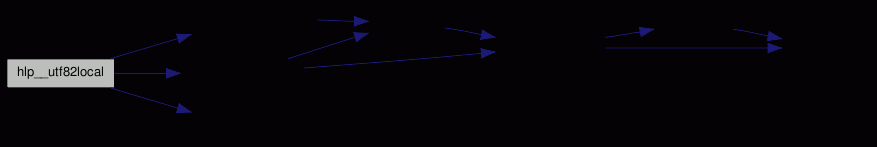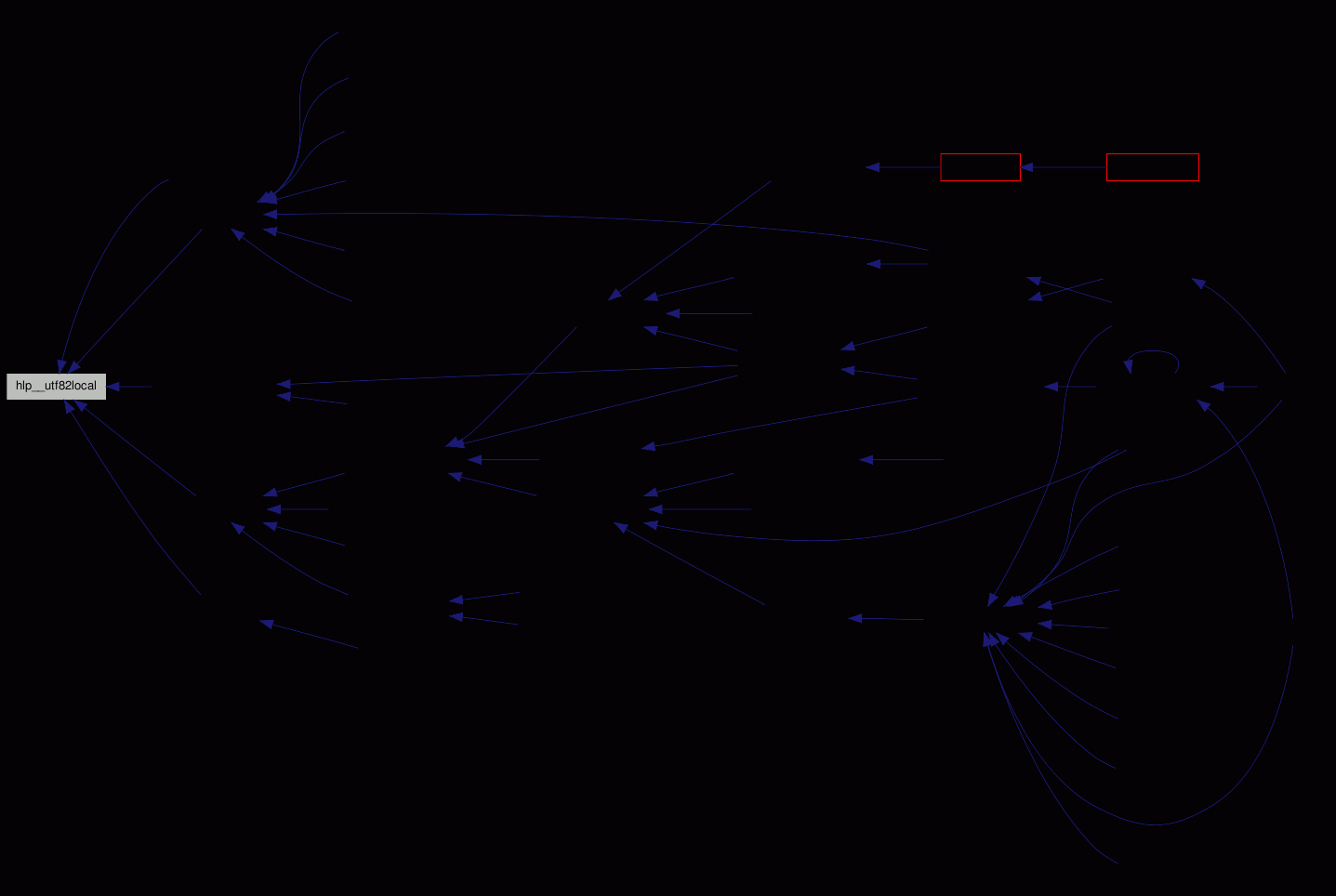Generated for fsvs by1.5.9